# ISSN 1996-3955 ИФ РИНЦ = 0,580

### MICROELECTRONIC CONTROLLING OF REALTIME COMPLICATED TECHNICAL SYSTEMS

The very important parameter of high-speed realtime automation facilities is simplicity and reliability of control subsystems. For classical structure of Moore micro-programmed automatons (MPA), the operation algorithms are described as follows: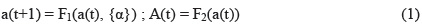where a(t) and a(t+1) are present (t) and following (t+1) state of the automaton; {α} - α1, α2,...αq - logical preconditions; А(t) - A1, A2,...Ak -MPA output commands; F1 and F2 - Boolean function systems of MPA transitions and outputs.

Fig. 1 depicts a scheme of the automaton corresponding to equations (1); fig. 2 - control algorithm flow-graph (AFG) and fig. 3 - transition graph of Moore automaton. Algorithm of transition from AFG to the graph is presented in fig. 1. For simple MPA with number of states ≤32 and number of logical preconditions q≤5, capacity of programmable read only memory (PROM) does not exceed 5 kbit. Memory capacity (V) required for realization of Boolean function systems of transitions F1 shall be as V(F)=m2m+q, where: m - length of representation code a(t) and a(t+1). When realizing more complicated automatons: with m=5, q=10, PROM=160kbit and m=6, q=16, PROM =24Мbit. Such memory capacity is too large for high-speed realtime automatons.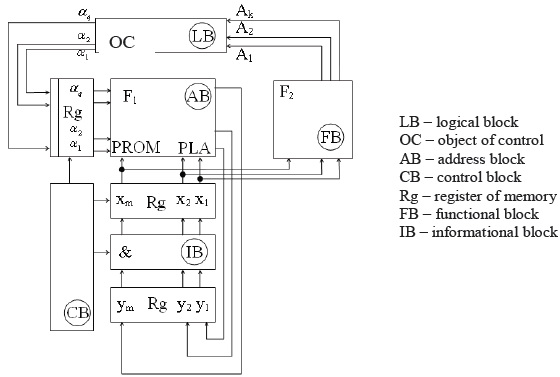Figure 1. Structural scheme of Moore automaton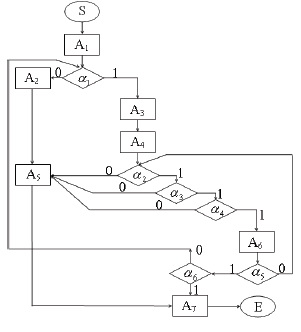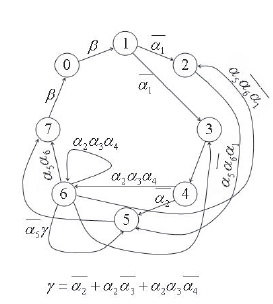Figure 2. Flow-graph of control algorithm             Figure 3. Graph turning Moore automaton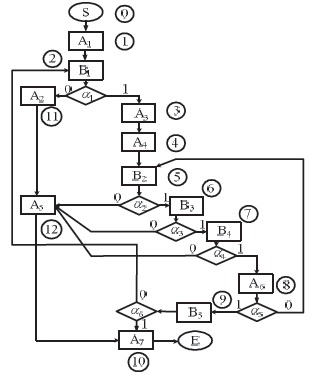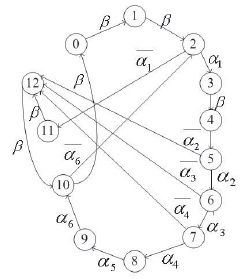Figure 4. Flow-graph of new control algorithm                   Figure 5. Graph-turning new automaton

To reduce complexity of F1 we may switch to realization by means of programmable logic arrays (PLA) or programmable logic devices (PLD) applying science intensive arrangements to minimize Boolean functions (fig. 5, 6). However for complicated automatons if m=5 and q=10 the effect from minimization is under 20% and approximates to only a few percents. The second way to structural optimization of PROM is application of decomposition method i.e. partitioning of complicated automatons into a set of simple MPAs (fig. 4).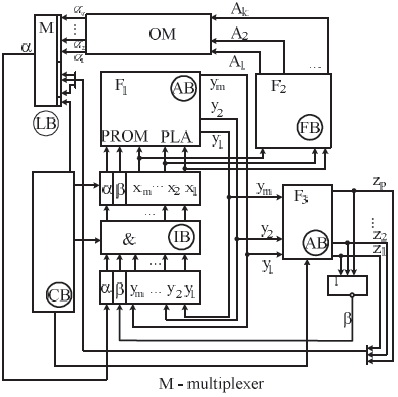Figure 6. Structural scheme of new automaton

We introduce a new method of complicated MPAs synthesis (fig. 1, 2) which is based on transformation of the specified algorithm flow-graph (AFG). A void action operator Ak+1 should be inserted after each logical operator αi in case when output of this αi is linked with the following αj without intermediate operator Ap (p - 1, 2, ..., k).

A void operator is also inserted before the αi to which control is transferred from several action operators. The modified AFG (fig. 4) allows us to get the transitions graph (fig. 5) in which there are two types of links of a(t) and a(t+1): unconditional β and conditional on single αj, number (j) of which is completely specified by a(t) code.

Formal description of the new MPA is made by the following equations: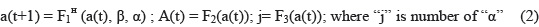The length (p) of code z1, z2,..., zp of representation "j" is defined as p=loq2q. The structural scheme of the MPA (fig. 3) corresponding to equations (2) is presented in fig. 6. As appears from (2), the new system of Boolean functions (F1н) of MPA address part does not depend on number (q) of logical conditions, so V(F1н)=m2m+2. In case (m=5, q=10) we have L= V(F1)/ V(F1н) = 256; and for (m=6, q=16) we have L= 214≈ 16,000.

Modification of AFG does not break the automaton operation algorithms, but the realization is carried out by MPA structure with much less memory capacity of address part in some hundreds or even thousands times. It is significant that the new structure of MPA will be as efficient in case of realization by PLA or PLD since the number of input variables for F1н always equals (m+2); and for practical even the most complicated AFG: m+2≤8. As for systems that are not purposed for real-time information processing, instead of hardware realization of MPA by PROM, PLA and PLD we can switch to software realization by microcontroller. For classical structure with (m+q)≥12 this is problematic, but for the new structure of MPA applying (m+q)≤8, realization by eight-bit microcontroller is performed by direct reading method.

Thus the new method of MPA synthesis is a breakthrough in the problem of creation of reliable high-speed control facilities for complicated technical systems.

References

1.   Mukhopad Yu.F. Theory discrete device. Irkutsk. Yniversitet of Transport, Russia, 2009. - 149 p.

2.   Mukhopad A. Yu. Mukhopad. Yu.F. Method of the syntheses complex automats. Novosibirsk. Scientific lead. NSTU, issue 1(34), 2009. p219 -222.

3.   Mukhopad A. Yu. Mukhopad. Yu.F. Microprogramme avtomat. Patent RU useful model № 064785 from 15.12.2008.

4.   Mukhopad. Yu.F. Badmaeva T.S. Synthes automats on decomposition scheme of the algorithm. // Information systems of the control and management on transport. Irkutsk. Yniversitet of Transport, 2002. p14-25.

5.   Ritsar B.E., Kmet A.B. New method of the syntheses digital device on PLA. Cybernetic and system analys. 2003, №2. p 63-89.

6.   Solovyov V.V., Klimovich A. Logical designing the digital systems on PLD. Moscow, Hot line-Telecom, 2008. -375 p.

7.   Nim Wilmshurst. Desiging embedded systems with PIC microcontrollers. Elsevier Ltd 2007, Boulevard, Langford Lane, Kidlington, Oxford, OX5 1GB, UK, 543 p.

### Библиографическая ссылка

Mukhopad Yu.F., Yu Mukhopad. A. MICROELECTRONIC CONTROLLING OF REALTIME COMPLICATED TECHNICAL SYSTEMS // Международный журнал прикладных и фундаментальных исследований. – 2009. – № 2. – С. 26-29;
URL: http://applied-research.ru/ru/article/view?id=360 (дата обращения: 20.01.2021).

Предлагаем вашему вниманию журналы, издающиеся в издательстве «Академия Естествознания»
(Высокий импакт-фактор РИНЦ, тематика журналов охватывает все научные направления)

«Фундаментальные исследования» список ВАК ИФ РИНЦ = 1.074